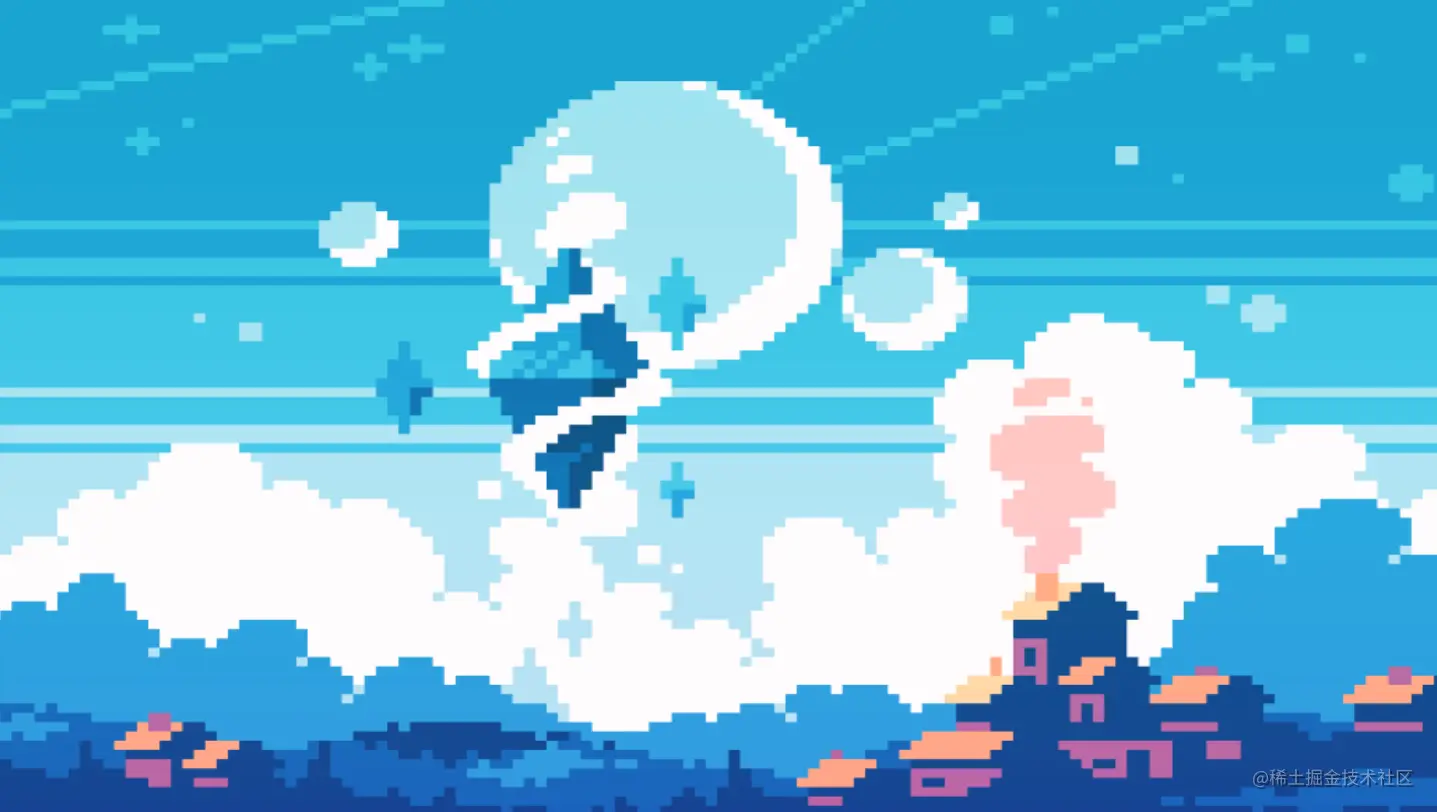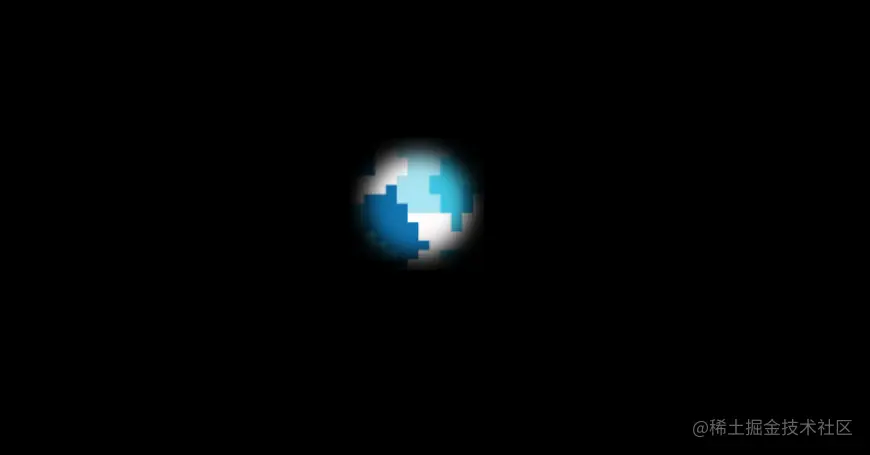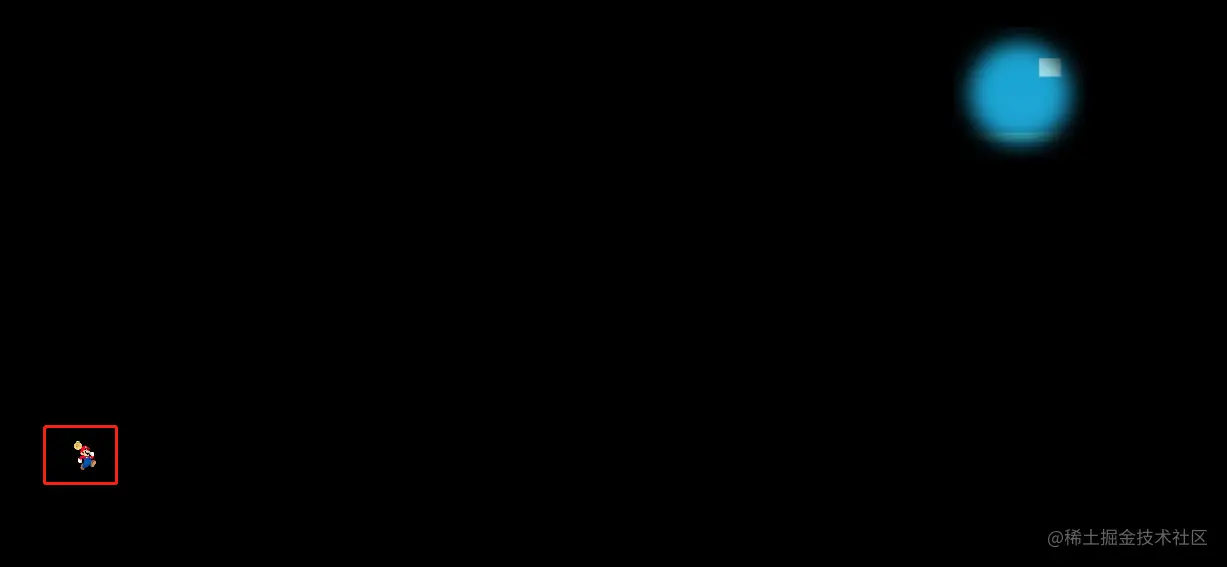# 基于PixiJs实现的简单小游戏

### 游戏实现所需要准备的前置知识

• `PixiJs`的应用和舞台
• `PixiJs`的纹理缓存
• `PixiJs`的精灵
• `PixiJs`的图形绘制类
• `PixiJs`的滤镜
• `PixiJs`的遮罩
• `PixiJs`的容器
• `PixiJs`的事件系统
• 碰撞检测算法

### `PixiJs`的应用和舞台

``````// 创建应用
const app = new PIXI.Application();
document.body.appendChild(app.view);
// 舞台
console.log(app.stage)
``````

### `PixiJs`的纹理缓存

tips1 通过`app.loader.add`加载图像或纹理贴图时，可以指定别名，别名需要全局唯一。接下来使用的时候可以通过指定的别名来找到纹理缓存

tips2 `app.loader.add`可以链式调用添加多个纹理缓存

``````app.loader.add('grass', 'https://pic.qy566.com/pixijs/images/bg.jpg')
``````

### `PixiJs`的精灵

• 通过单个图像文件
• 通过雪碧图创建（与css里的雪碧图用法一致）
• 通过纹理贴图实现 （需要特定的应用来生成图片与对应的描述json文件）

``````const background = new PIXI.Sprite(resources.grass.texture);
background.width = app.screen.width;
background.height = app.screen.height;
``````### `PixiJs`的图形绘制类

``````// 圆的大小

// 圆的边缘模糊的大小
const blurSize = 16;
// 绘制圆形填充红色
const circle = new PIXI.Graphics()
.beginFill(0xFF0000)
.endFill();
``````

### `PixiJs`的滤镜

``````// 高斯模糊滤镜
circle.filters = [new PIXI.filters.BlurFilter(blurSize)];
// 创建大小是圆形2倍的矩形区域
const bounds = new PIXI.Rectangle(0, 0, (radius + blurSize) * 2, (radius + blurSize) * 2);
// 创建以圆形作为填充的通用纹理
const texture = app.renderer.generateTexture(circle, PIXI.SCALE_MODES.NEAREST, 1, bounds);
// 生成精灵
const focus = new PIXI.Sprite(texture);
``````

### `PixiJs`的遮罩

``````background.mask = focus;
``````### `PixiJs`的容器

``````const captain = new PIXI.Sprite(resources.captain.texture);
captain.width = 30;
captain.height = 30;
captain.position.x = Math.floor(Math.random() * app.screen.width)
captain.position.y = Math.floor(Math.random() * app.screen.height)
``````### `PixiJs`的容器

`PixiJs`的容器的容器概念类似于`Ps`里的分组的概念，将某些精灵，聚合为一个视为一种不包含纹理的特殊精灵。那现在我们就需要修改一下我们的代码

• 创建容器
``````let containers = new PIXI.Container();
``````
• 将背景添加到容器内
``````- app.stage.addChild(xx);
``````
• 将要寻找的元素添加到容器
``````- app.stage.addChild(captain);
``````
• 将容器添加到舞台
``````+ app.stage.addChild(containers);
``````
• 为容器添加遮罩
``````- background.mask = focus;
``````

### `PixiJs`的事件系统

``````app.stage.interactive = true;
app.stage.on('mousemove', pointerMove);
function pointerMove(event) {
focus.position.x = event.data.global.x - focus.width / 2;
focus.position.y = event.data.global.y - focus.height / 2;
}
``````

tips1 这里`app.stage.interactive = true;` 是关键，只有设置了`interactive`的容器才会响应dom事件

### 碰撞检测算法

``````function hitTestRectangle(r1, r2) {

//Define the variables we'll need to calculate
let hit, combinedHalfWidths, combinedHalfHeights, vx, vy;

//hit will determine whether there's a collision
hit = false;

//Find the center points of each sprite
r1.centerX = r1.x + r1.width / 2;
r1.centerY = r1.y + r1.height / 2;
r2.centerX = r2.x + r2.width / 2;
r2.centerY = r2.y + r2.height / 2;

//Find the half-widths and half-heights of each sprite
r1.halfWidth = r1.width / 2;
r1.halfHeight = r1.height / 2;
r2.halfWidth = r2.width / 2;
r2.halfHeight = r2.height / 2;

//Calculate the distance vector between the sprites
vx = r1.centerX - r2.centerX;
vy = r1.centerY - r2.centerY;

//Figure out the combined half-widths and half-heights
// 修正滤镜产生的blur量
combinedHalfWidths = r1.halfWidth + r2.halfWidth - 20;
combinedHalfHeights = r1.halfHeight + r2.halfHeight - 20;

//Check for a collision on the x axis
if (Math.abs(vx) < combinedHalfWidths) {
if (Math.abs(vy) < combinedHalfHeights) {
hit = true;
} else {
hit = false;
}
} else {

hit = false;
}

return hit;
};
``````

### 获胜条件

``````if (hitTestRectangle(captain, focus)) {
clearTimeout(timer)
document.getElementById('time').innerHTML = '总用时'+ t + '秒'